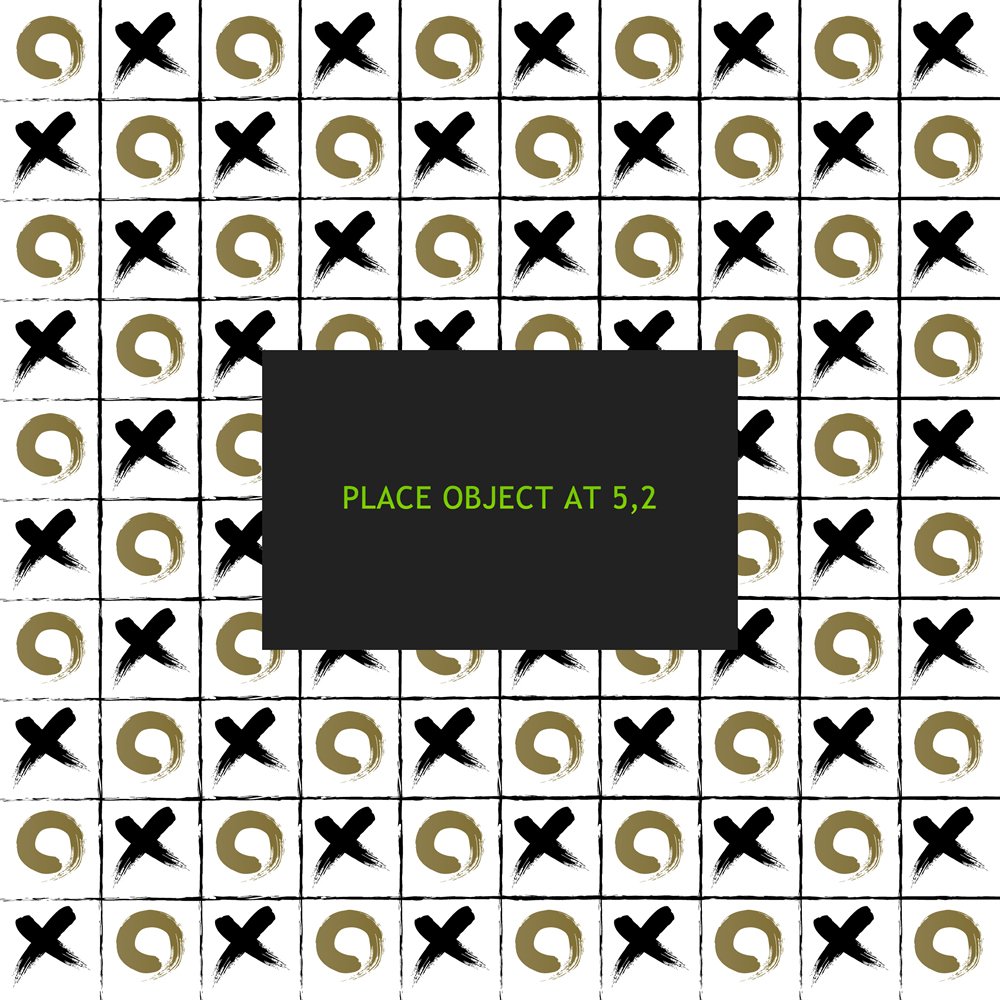# Using an Alignment Grid

### Why I’m using an Alignment Grid

I’ve been writing a lot of Phaser Code for an app for my company. I’ve been using percentages of the game’s width to be able to place the elements. This does work but it has been very time consuming to put together pop-up screens quickly. I needed to come up with something quicker and easier to use. Now you can use this method for placing sprites in a game, but it is most useful for placing static elements like buttons and text.

### The Old Way Using X and Y

When I was using Flash, or any of my old software meant for developing for desktop I could simply place an element at an x and y coordinate and that would be fine. The problem lies in with mobile devices all being different sizes. I could place an element at an x position of 450 and it would be at different places on different devices.

### I still need my X and Y!

I need a coordinate system that is standard across all devices and need to be able to use an x and y position system.

### The Align Grid Code

This test code will make a grid 3 columns by 9 rows and place the 3 ducks at random rows.

If using chrome check different mobile sizes, and you’ll see there is a consistent layout regardless of screen size.

### Using The Code

```var StateMain = {
},
create: function() {
//make an align grid
var grid = new AlignGrid(3, 9);
//turn on the lines for testing
//and layout
grid.show();
for (var i = 0; i < 3; i++) {
var duck = game.add.sprite(0, 0, "duck");
duck.anchor.set(0.5, 0.5);
//pick a random row
var yy = game.rnd.integerInRange(0, 8);
//place the duck at i for the column
//and yy as the random row
grid.placeAt(i, yy, duck);
}
},
update: function() {}
}```

### The AlignGrid Class

```class AlignGrid extends Phaser.Group {
constructor(cols = 3, rows = 3, par = null) {
super(game);
//if not parent is passed then use the game
if (par == null) {
par = game;
}
//cw cell width is the parent width divided by the number of columns
this.cw = par.width / cols;
//ch cell height is the parent height divided the number of rows
this.ch = par.height / rows;
//promote to a class variable
this.par = par;
}
//place an object in relation to the grid
placeAt(xx, yy, obj) {
//calculate the center of the cell
//by adding half of the height and width
//to the x and y of the coordinates
var x2 = this.cw * xx + this.cw / 2;
var y2 = this.ch * yy + this.ch / 2;
obj.x = x2;
obj.y = y2;
}
//mostly for planning and debugging this will
//create a visual representation of the grid
show() {
this.graphics.lineStyle(4, 0xff0000, 1);
//
//
for (var i = 0; i < this.par.width; i += this.cw) {
this.graphics.moveTo(i, 0);
this.graphics.lineTo(i, this.par.height);
}
for (var i = 0; i < this.par.height; i += this.ch) {
this.graphics.moveTo(0, i);
this.graphics.lineTo(this.par.width, i);
}
}
}```